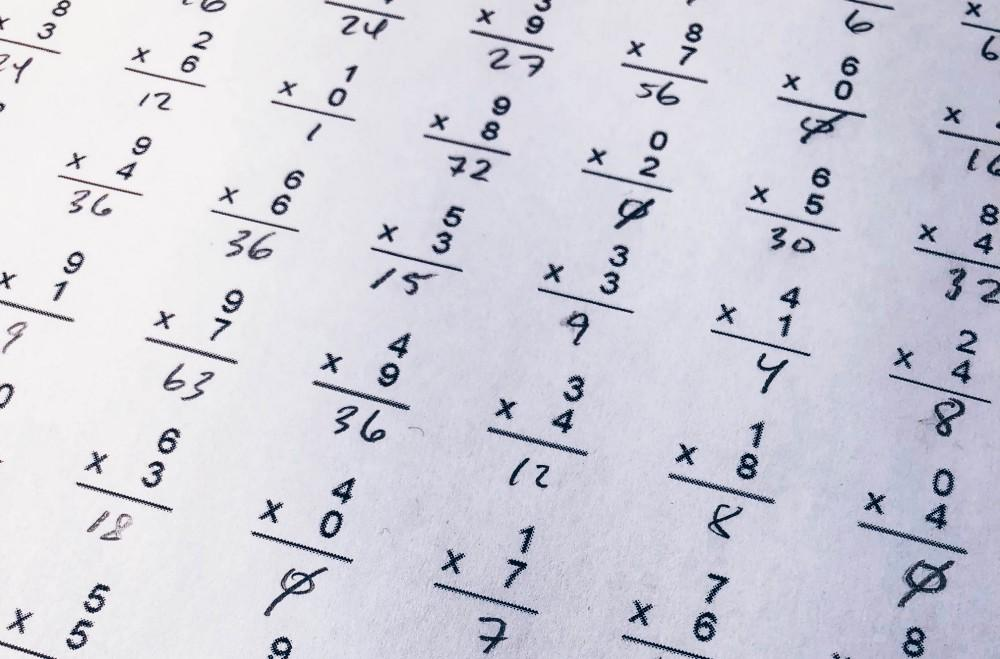## 为什么要学数学？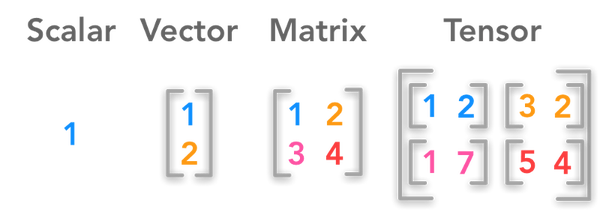标量、向量、矩阵、张量；图片来源：hadrienj.github.io

## 标量

``````import numpy as np
np.ScalarType``````

``````(int,
float,
complex,
int,
bool,
bytes,
str,
memoryview,
numpy.bool_,
numpy.int8,
numpy.uint8,
numpy.int16,
numpy.uint16,
numpy.int32,
numpy.uint32,
numpy.int64,
numpy.uint64,
numpy.int64,
numpy.uint64,
numpy.float16,
numpy.float32,
numpy.float64,
numpy.float128,
numpy.complex64,
numpy.complex128,
numpy.complex256,
numpy.object_,
numpy.bytes_,
numpy.str_,
numpy.void,
numpy.datetime64,
numpy.timedelta64)``````

``````a = 5
b = 7.5
print(type(a))
print(type(b))
print(a + b)
print(a - b)
print(a * b)
print(a / b)``````

``````<class 'int'>
<class 'float'>
12.5
-2.5
37.5
0.6666666666666666``````

``````import numpy as np

def isscalar(num):
if isinstance(num, generic):
return True
else:
return False

print(np.isscalar(3.1))
print(np.isscalar([3.1]))
print(np.isscalar(False))``````

``````True
False
True``````

## 向量

``````x = [1, 2, 3]
y = [4, 5, 6]

print(type(x))``````

``<class 'list'>``

`+`并不表示向量的加法，而是列表的连接：

``print(x + y)``

``[1, 2, 3, 4, 5, 6]``

``````z = np.add(x, y)
print(z)
print(type(z))``````

``````[5 7 9]
<class 'numpy.ndarray'>``````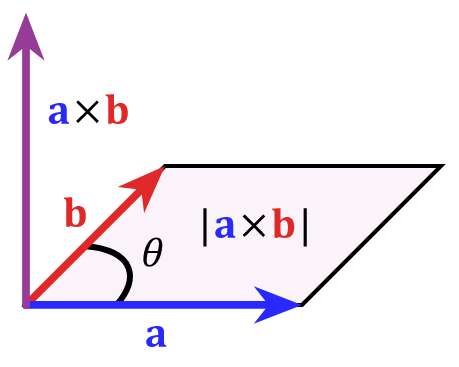图片来源：维基百科
``np.cross(x, y)``

``[-3  6 -3]``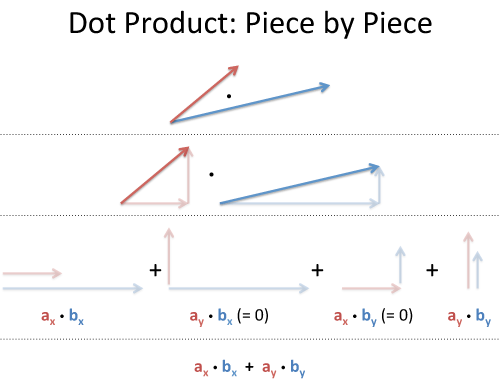图片来源：betterexplained.com
``np.dot(x, y)``

``32``

## 矩阵

m x n可表示为以下形式：

``````x = np.matrix([[1,2],[3,4]])
x``````

``````matrix([[1, 2],
[3, 4]])``````

``x.mean(0)``

``matrix([[2., 3.]]) # (1+3)/2, (3+4)/2``

``x.mean(1)``

``````z = x.mean(1)
z``````

``````matrix([[1.5],   # (1+2)/2
[3.5]])  # (3+4)/2``````

`shape`属性返回矩阵的形状：

``z.shape``

``(2, 1)``

``np.shape([1, 2, 3])``

``(3,)``

``np.shape(1)``

``()``

``````x = np.matrix([[1, 2], [4, 3]])
x.sum()``````

``10``

``````x = np.matrix([[1, 2], [4, 3]])
x + 1``````

``````matrix([[2, 3],
[5, 4]])``````

``````x * 3
``````

``````matrix([[ 3,  6],
[12,  9]])``````

``````x = np.matrix([[1, 2], [4, 3]])
y = np.matrix([[3, 4], [3, 10]])``````

x和y的形状均为`(2, 2)`

``x + y``

``````matrix([[ 4,  6],
[ 7, 13]])``````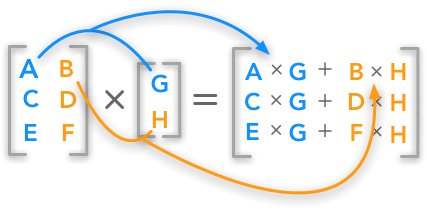图片来源：hadrienj.github.io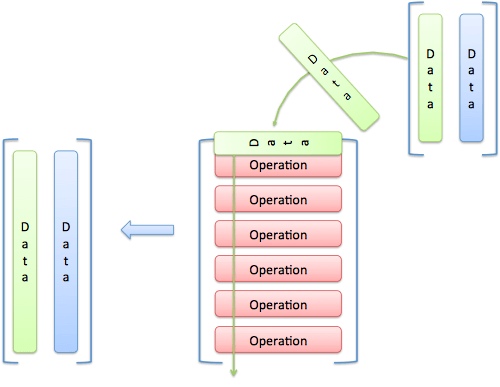图片来源：betterexplained.com
``````x = np.matrix([[1, 2], [3, 4], [5, 6]])
y = np.matrix([, ]

x * y``````

``````matrix([[ 33],
[ 73],
])``````

``````x = np.matrix([[1, 2], [3, 4], [5, 6]])
x``````

``````matrix([[1, 2],
[3, 4],
[5, 6]])``````

``x.transpose()``

``````matrix([[1, 3, 5],
[2, 4, 6]])``````

## 张量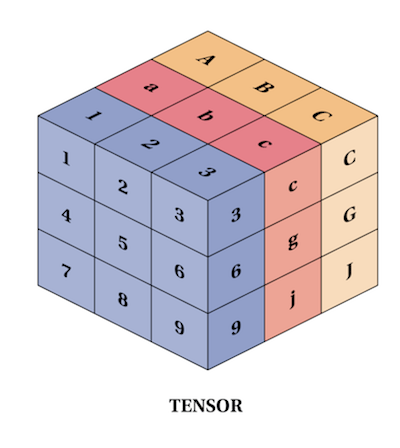图片来源：refactored.ai

``````import numpy as np

t = np.array([
[[1,2,3], [4,5,6], [7,8,9]],
[[11,12,13], [14,15,16], [17,18,19]],
[[21,22,23], [24,25,26], [27,28,29]],
])

t.shape``````

``(3, 3, 3)``

``````s = np.array([
[[1,2,3], [4,5,6], [7,8,9]],
[[10, 11, 12], [13, 14, 15], [16, 17, 18]],
[[19, 20, 21], [22, 23, 24], [25, 26, 27]],
])

s + t``````

``````array([[[ 2,  4,  6],
[ 8, 10, 12],
[14, 16, 18]],

[[21, 23, 25],
[27, 29, 31],
[33, 35, 37]],

[[40, 42, 44],
[46, 48, 50],
[52, 54, 56]]])``````

`s * t`得到的是阿达马乘积（Hadamard Product），也就是分素相乘（element-wise multiplication），将张量s和t中的每个元素相乘，所得乘积为结果张量对应位置的元素。

``s * t``

``````array([[[  1,   4,   9],
[ 16,  25,  36],
[ 49,  64,  81]],

[[110, 132, 156],
[182, 210, 240],
[272, 306, 342]],

[[399, 440, 483],
[528, 575, 624],
[675, 728, 783]]])``````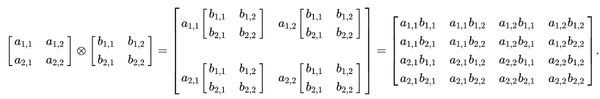图片来源：维基百科

``````s = np.array([[[1, 2], [3, 4]]])
t = np.array([[[5, 6], [7, 8]]])

np.tensordot(s, t, 0)``````

``````array([[[[[[ 5,  6],
[ 7,  8]]],
[[[10, 12],
[14, 16]]]],
[[[[15, 18],
[21, 24]]],
[[[20, 24],
[28, 32]]]]]])``````

``torch.ones(5, 5)``

``````tensor([[ 1.,  1.,  1.,  1.,  1.],
[ 1.,  1.,  1.,  1.,  1.],
[ 1.,  1.,  1.,  1.,  1.],
[ 1.,  1.,  1.,  1.,  1.],
[ 1.,  1.,  1.,  1.,  1.]]``````